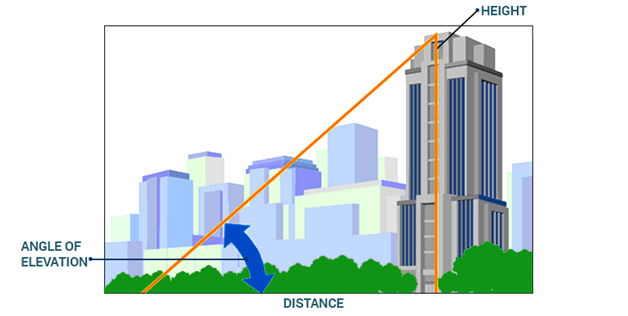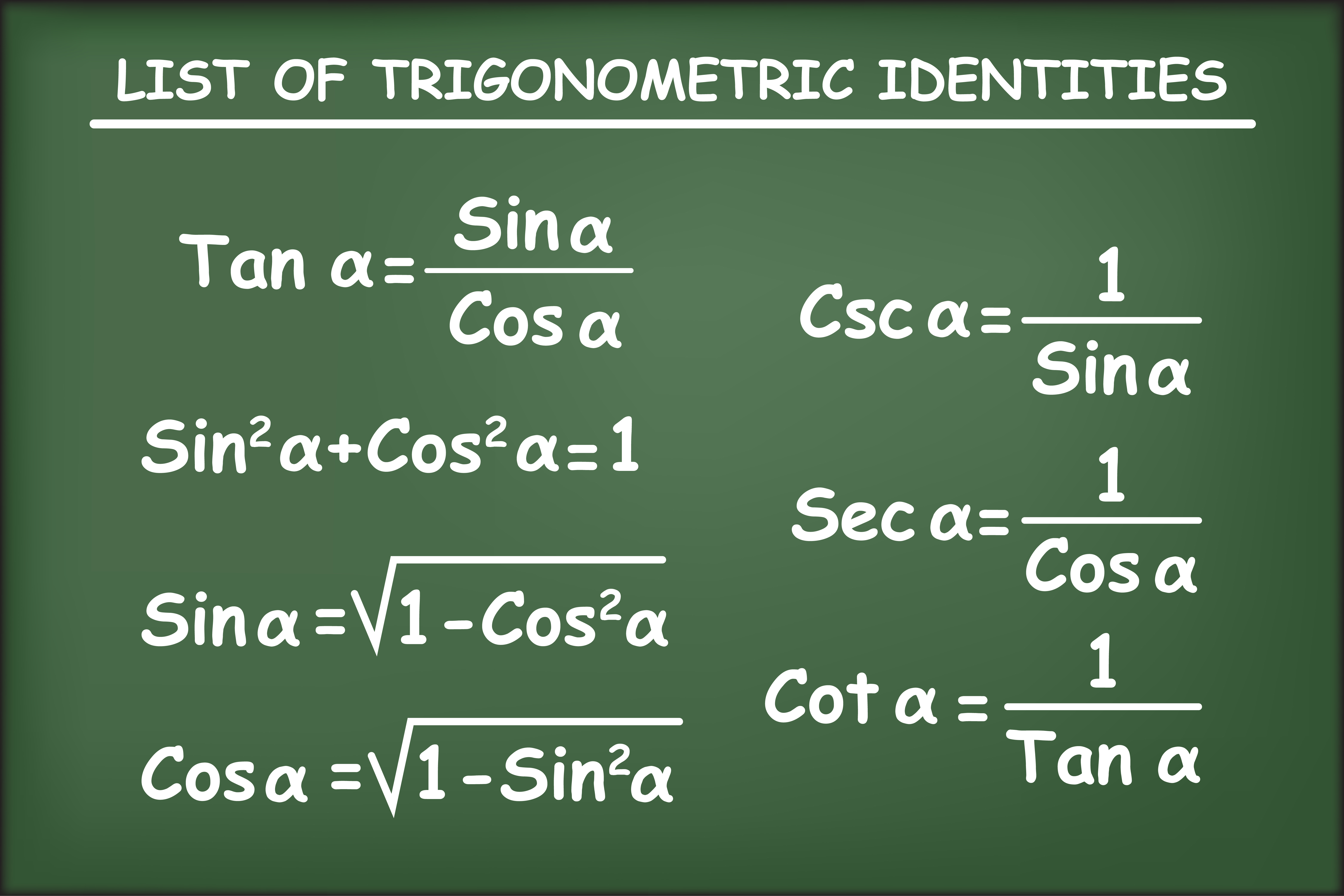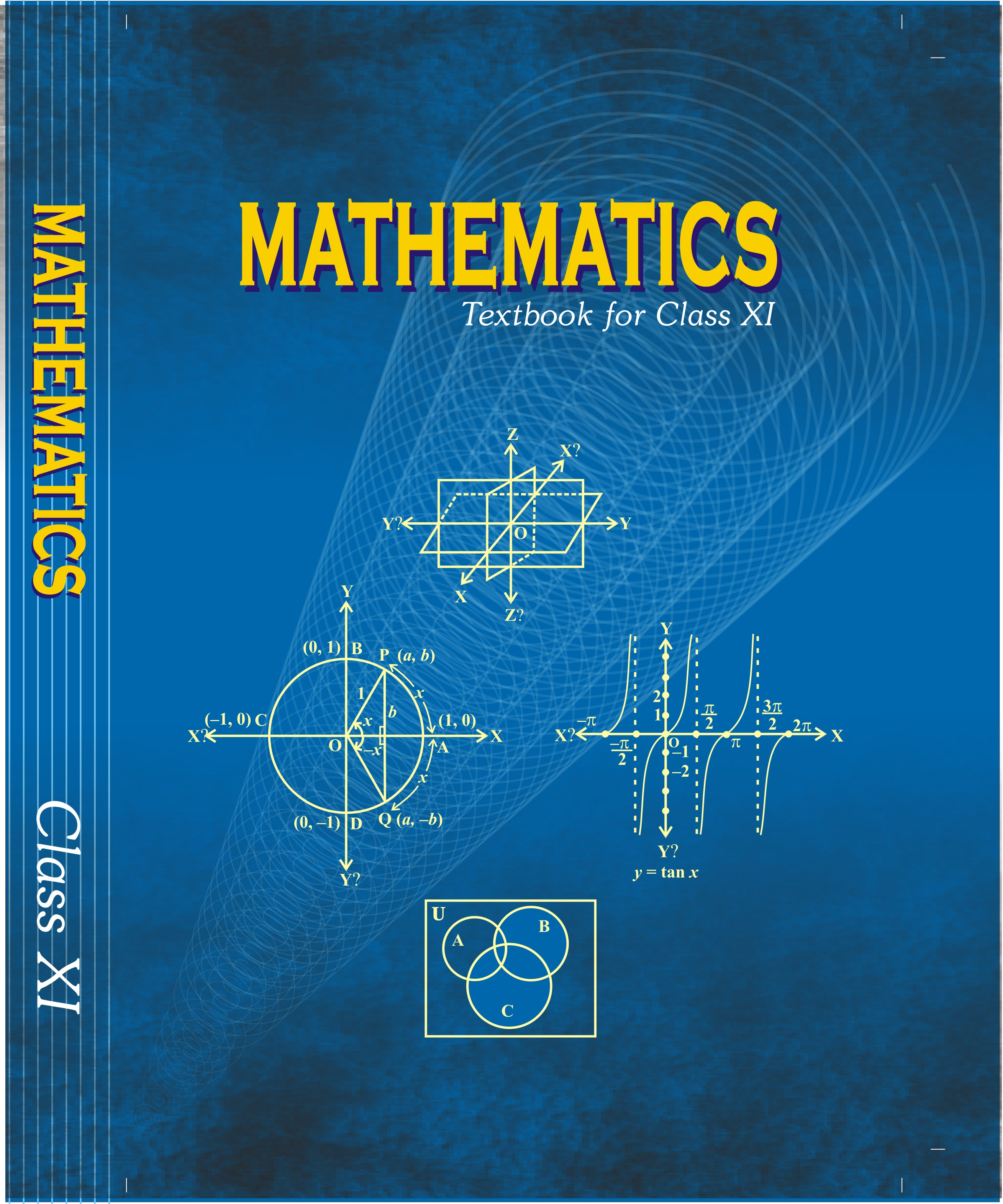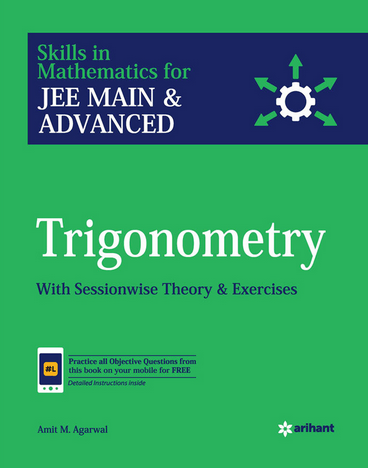# Trigonometry   Share

## What is Trigonometry

Trigonometry is defined as trigonon means triangle and metron means measurement or we can say trigonometry means the measurement of a triangle. A triangle is divided into six parts, 3-sides and 3-angles so to determine the relationship between sides and angles we need to study trigonometry. In JEE Mains or other engineering exams, trigonometry is the foundation of all the chapters. Every year you see at least 2-4 questions in those examinations. But this was the only data of direct questions from this chapter and will you imagine that in 20-25% questions the concept of trigonometry is used. You are very well familiar with the basics of trigonometry as you already studied in previous classes now in this chapter you will learn interesting facts about trigonometry. The whole chapter revolves around three basic terms sine, cosine, and tangent including three parameters (a,b,c) or we can say sides of the triangle.

If we talk about the importance of this chapter, you will find that how questions can be tricked using trigonometry in Calculus, Matrices and Determinant, and Functions. Overall this chapter is directly or indirectly present in your whole textbook. So, you need to do proper practice, you should solve an ample amount of questions and make sure you are good with calculations.

## Why Trigonometry:

Let us take a real-life example of Trigonometry,

In summer if you are out from your home, you always try to keep yourself within the shadow ( a tree or a building) but have you ever observed that in day time the shadow is small in size and at morning or evening shadow is large in size why this is happening, the size of building or tree is not changing even the sun's size is not changing?

The reason we studied or known to us is that the position of the sun is changing with respect to the earth.

Prepare Online for JEE Main/NEET

Crack JEE 2021 with JEE/NEET Online Preparation Program

Here the change in position is explained as the angle changes from 0º to 180º and at 90º the position of the sun is exactly above the object that's why their shadow becomes diminished and from 0º to 180º shadow starts first decreasing till 90º and starts increasing.

Let's take an application of TrigonometryIn the given figure if know the elevation angle and distance of the building you can easily calculate the height of the building using, .

## Trigonometry Notes

After studying Trigonometry you will able to:

1. Understand the trigonometry identities.
2. Resolve the problem based on periodic functions.
3. Solve problem-based on Inverse trigonometry.

Important Topics:

1. Trigonometry Function and their Identities
2. Trigonometry Equation
3. Inverse Trigonometry

## Overview of ChapterTrigonometry Function and their Identities: In this topic, we learn a small and very important part of trigonometry, as every function have both a domain and a range so here we will study the range and domain of every trigonometry function and their behavior. The chapter explains all the identities formed with these functions. It is the first step to understanding trigonometry, and finally, multi-concept problems can be solve using these concepts.

Trigonometry Equation: This topic involves solving trigonometric equations, general solutions to all the trigonometric functions. This chapter has a lot of properties that are important to remember. The chapter contains the application of concepts that you learned in the previous chapters of trigonometry. If your basic is clear then there will be no issue for you to be perfect in this section.

• cos (α - β) = cos α cos β + sin α sin β
• cos (α - β) = cos α cos β - sin α sin β
• sin (α - β) = sin α cos β - cos α sin β
• sin (α + β) = sin α cos β + cos α sin β
•

Some Important General Solutions of Equations

Inverse Trigonometric functions: This chapter is very different from the above chapters and forms a greater part of trigonometry. In the above topic, you study the trig function associated angle with but in this topic, you will study angles associated with trig function. In simple words, it contains the inverse of the functions that you were learned in the first lesson.

## How to prepare Trigonometry

• The first step is to clear all your basics from previous classes.
• In NCERT all the formulas or identities are well derived so first observe those and try to derive it on your own and you will learn more new things.
• Domain and Range is the important part of trigonometry function so to remember it plot these graph by on your own.
• Inverse trigonometry may be tough for you if you study first time, but more and more times you study you keep on learning the basics and finally it becomes easy for you.
• NCERT should be your first book to learn this chapter after this you can solve any other textbooks.

## Tips that will help you in preparing trigonometry in the best possible way:

• Always try to solve questions using a different approach.

For eg. \tan\theta=\frac{sin\theta}{cos\theta}\;\;\text{or,}\;\tan\theta=\frac{1}{cot\theta}

• Trigonometry starts with a triangle so keep in your mind that behind every question there is a triangle.
• If you are going for an objective approach than you can put   or u can verify your answer by this, but always keep in your mind that it is not hard and fast rule that always be true.

## Best books for the preparation of Trigonometry

First, finish all the concepts, example and questions given in NCERT Maths Book along with Miscellaneous Exercise. You must be thorough with the concept of Trigonometry. Then you can refer to the book Algebra by Dr. SK goyal or RD Sharma but make sure you follow any one of these not all. Trigonometry is explained very well in these books and there are an ample amount of questions with crystal clear concepts. Choice of reference book depends on person to person, find the book that best suits you the best, depending on how well you are clear with the concepts and the difficulty of the questions you require.

## Maths Chapter-wise Notes for Engineering exams

 Chapters Chapters Name Chapter 1 Sets, Relations, and Functions Chapter 2 Complex Numbers and Quadratic Equations Chapter 3 Matrices and Determinants Chapter 4 Permutations and Combinations Chapter 5 Binomial Theorem and its Simple Applications Chapter 6 Sequence and Series Chapter 7 Limit, Continuity, and Differentiability Chapter 8 Integral Calculus Chapter 9 Differential Equations Chapter 10 Coordinate Geometry Chapter 11 Three Dimensional Geometry Chapter 12 Vector Algebra Chapter 13 Statistics and Probability Chapter 15 Mathematical Reasoning Chapter 16 Mathematical Induction

### Topics from Trigonometry

• Trigonometrical functions, Inverse trigonometrical functions and their properties ( AEEE, JEE Main, TS EAMCET, AP EAMCET, COMEDK UGET ) (120 concepts)
• Trigonometrical identities and equations ( AEEE, JEE Main, TS EAMCET, AP EAMCET, COMEDK UGET ) (269 concepts)
• Height and distance ( AEEE, JEE Main, TS EAMCET, AP EAMCET, COMEDK UGET ) (16 concepts)
• Trigonometric Ratios and Transformation Formulas ( AEEE, JEE Main, TS EAMCET, AP EAMCET, COMEDK UGET ) (60 concepts)
• Trigonometric Functions ( AEEE, JEE Main, TS EAMCET, AP EAMCET, COMEDK UGET ) (36 concepts)
• Trigonometric Equations ( AEEE, JEE Main, TS EAMCET, AP EAMCET, COMEDK UGET ) (32 concepts)
• Properties and Solutions of Triangle ( AEEE, JEE Main, TS EAMCET, AP EAMCET, COMEDK UGET ) (56 concepts)
• Inverse Trigonometric Functions ( AEEE, JEE Main, TS EAMCET, AP EAMCET, COMEDK UGET ) (81 concepts)

### Important Books for Trigonometry

•••Exams
Articles
Questions